# In the Spirit of Giving Tuesday, Donate to the Annual Appeal

## A Global Day of Giving

#GivingTuesday connects diverse groups of individuals, communities, and organizations around the world for one common purpose: to celebrate and encourage giving.

#GivingTuesday is about ordinary people coming together to do extraordinary things. Make a donation to the Annual Appeal and you will help us do extraordinary things for your student and Northside!

## You don't have to wait until Tuesday, donate today!

We still have a long way to go to reach our goal of 100% participation by NCP families and \$500,000!

Employer donations can double or triple the impact of your donation. That means your \$50 donation can result in up to \$150 for Northside! If you aren't sure if your company matches employee contributions, contact your human resources department.

We still have a long way to go. Don't delay! Please donate today.

Keep Northside strong! All donations are appreciated!

 table div table+table+table+table+table+table div table{width:100%;padding:0}table div table+table+table+table+table+table div table img{width:96.23%;padding:0;float:none}table div table+table+table+table+table+table div table td{width:100%;padding:0 1.88% 18px}/* styles */# Spring Break 2020 Trip to Costa Rica

Informational meeting
Monday, December 2
6:00 pm
Room 233

Want to zip line through beautiful Costa Rica and learn about sustainable projects? Click HERE for an informational flyer.

Contact Ms. Torres prior to the meeting. Space is limited!

 table div table+table+table+table+table+table+table+table div table{width:100%;padding:0}table div table+table+table+table+table+table+table+table div table img{width:96.23%;padding:0;float:none}table div table+table+table+table+table+table+table+table div table td{width:100%;padding:0 1.88% 18px}/* styles */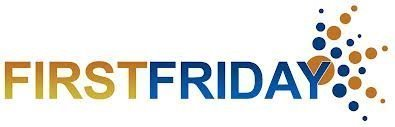Friday, December 6
8:00 - 10:00 am

Join fellow NCP parents at Friends of Northside's monthly coffee gathering. Stay for as long as your schedule allows.

 table div table+table+table+table+table+table+table+table+table+table div table{width:100%;padding:0}table div table+table+table+table+table+table+table+table+table+table div table img{width:96.23%;padding:0;float:none}table div table+table+table+table+table+table+table+table+table+table div table td{width:100%;padding:0 1.88% 18px}/* styles */# This Week in Sports

Please come out and cheer on our mighty Mustangs at the following games:

▪ Monday, December 2, 5:00 pm (V) vs. Rickover Naval at NCP
▪ Friday, December 6, 6:30 pm (V) Taft at Taft
▪ Saturday, December 7, 2:30 pm (V) Chicago Academy at TBD
▪ Monday, December 9, 5:00 pm (JV) and 6:30 pm (V) vs Maine East at Maine East
 ▪ Monday, December 2, 5:00 pm (V) vs. Rickover Naval at NCP
 ▪ Friday, December 6, 6:30 pm (V) Taft at Taft
 ▪ Saturday, December 7, 2:30 pm (V) Chicago Academy at TBD
 ▪ Monday, December 9, 5:00 pm (JV) and 6:30 pm (V) vs Maine East at Maine East

Men's and women's varsity and JV basketball schedules are posted on the MaxPreps website.

▪ Thursday, December 5, 5:00 pm (V) vs. Marshall at Marshall
▪ Monday, December 9, 5:00pm (V) vs. Beacon Academy at NCP
 ▪ Thursday, December 5, 5:00 pm (V) vs. Marshall at Marshall
 ▪ Monday, December 9, 5:00pm (V) vs. Beacon Academy at NCP

# Winter Sports Tryouts

## Winter Sports Online Registration is Now Open

All Winter student athletes must have their parents or guardian register them. Also, all students must have a current physical on file. Students need to turn in a copy of their current physical to their coach. Students can not participate in practice or games without a physical. Visit the NCP athletics website HERE and click on the registration tab.

Tryout Dates:

▪ Poms/Competitive Dance – TBA
▪ Men's Bowling – TBA
▪ Track (Men and Women) – Jan 2020
 ▪ Poms/Competitive Dance – TBA
 ▪ Men's Bowling – TBA
 ▪ Track (Men and Women) – Jan 2020

Visit the NCP Athletics website for information about Northside teams.

 table div table+table+table+table+table+table+table+table+table+table+table+table div table{width:100%;padding:0}table div table+table+table+table+table+table+table+table+table+table+table+table div table img{width:96.23%;padding:0;float:none}table div table+table+table+table+table+table+table+table+table+table+table+table div table td{width:100%;padding:0 1.88% 18px}/* styles */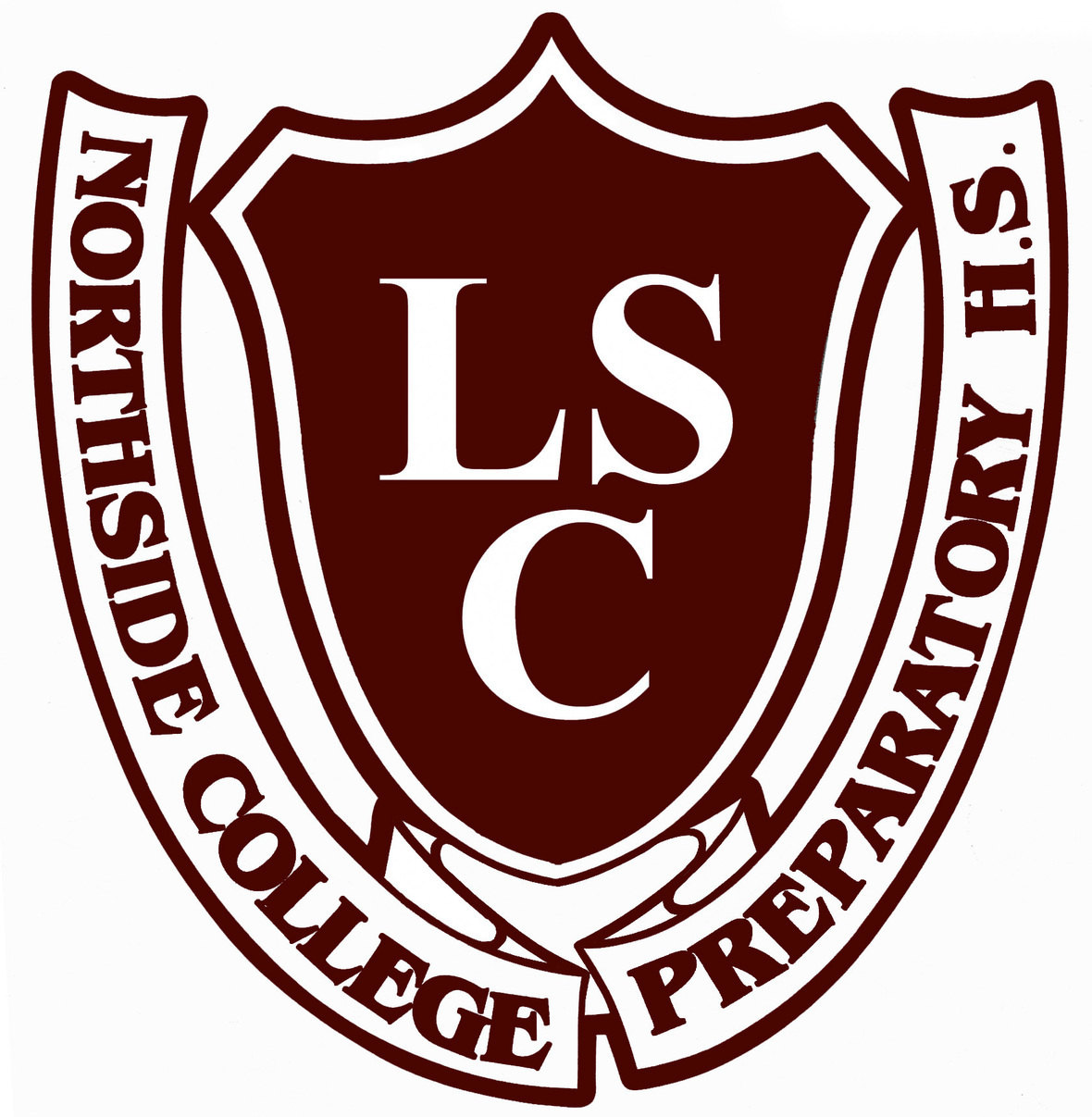# Local School Council (LSC) Meeting

Tuesday, December 17
6:30 pm
Faculty Lounge

The Local School Council (LSC) - the voice in the continuing evolution of Northside - is an elected body that plays an important role in the decision-making that affects our school. The LSC is responsible for approving the Continuous Improvement Work Plan (CIWP), principal selection and evaluation, and allocating discretionary funds to school programs.

LSC elections for six parent and two community representatives will be held in April 2020. Please attend upcoming LSC meetings to get a sense of what NCP’s Local School Council is working on this year.

 table div table+table+table+table+table+table+table+table+table+table+table+table+table+table div table{width:100%;padding:0}table div table+table+table+table+table+table+table+table+table+table+table+table+table+table div table img{width:96.23%;padding:0;float:none}table div table+table+table+table+table+table+table+table+table+table+table+table+table+table div table td{width:100%;padding:0 1.88% 18px}/* styles */# Cozy Winter Concert

Tuesday, December 17
7:00 pm
Auditorium

Please join the NCP Intermediate Strings, Concert Strings, and Chamber Strings for an evening concert.

 table div table+table+table+table+table+table+table+table+table+table+table+table+table+table+table+table div table{width:100%;padding:0}table div table+table+table+table+table+table+table+table+table+table+table+table+table+table+table+table div table img{width:96.23%;padding:0;float:none}table div table+table+table+table+table+table+table+table+table+table+table+table+table+table+table+table div table td{width:100%;padding:0 1.88% 18px}/* styles */# 2019 Teacher & Faculty Appreciation Lunch

Thursday, December 19

Show your appreciation for Northside’s wonderful teachers and staff by volunteering or donating an item for the annual luncheon. Sign up HERE to volunteer or to donate an item.

 table div table+table+table+table+table+table+table+table+table+table+table+table+table+table+table+table+table+table div table{width:100%;padding:0}table div table+table+table+table+table+table+table+table+table+table+table+table+table+table+table+table+table+table div table img{width:96.23%;padding:0;float:none}table div table+table+table+table+table+table+table+table+table+table+table+table+table+table+table+table+table+table div table td{width:100%;padding:0 1.88% 18px}/* styles */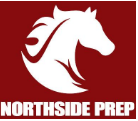# NCP Athletic Boosters Meeting

Thursday, December 19
7:00 pm
Faculty Lounge

The NCP Athletic Boosters seek to organize support and assistance for our exciting athletic programs, their coaches, and our amazing student-athletes. Please join us to share your ideas and concerns and help us in our quest to increase attendance and student participation at our team events. Get involved and celebrate the incredible achievements of our students outside of the classroom!

All parents and guardians are welcome. Go Mustangs!!!

 table div table+table+table+table+table+table+table+table+table+table+table+table+table+table+table+table+table+table+table+table div table{width:100%;padding:0}table div table+table+table+table+table+table+table+table+table+table+table+table+table+table+table+table+table+table+table+table div table img{width:96.23%;padding:0;float:none}table div table+table+table+table+table+table+table+table+table+table+table+table+table+table+table+table+table+table+table+table div table td{width:100%;padding:0 1.88% 18px}/* styles */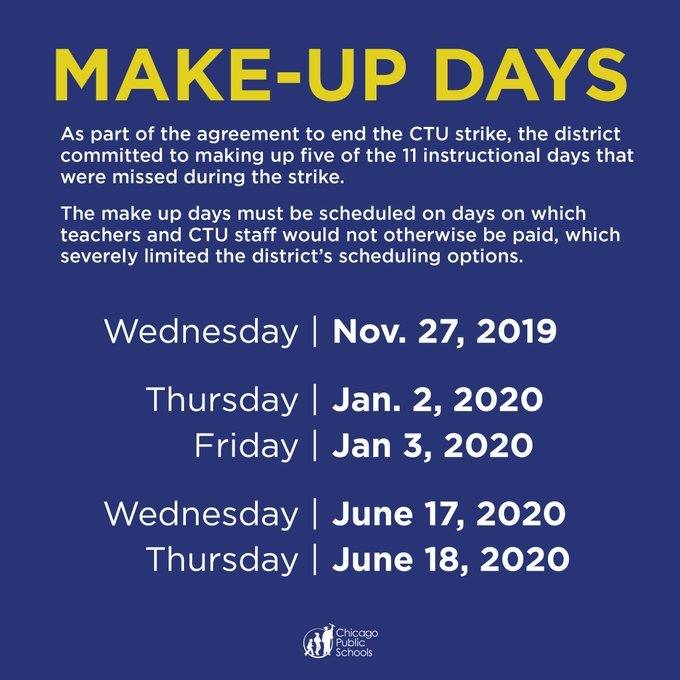# Make-Up Days

Chicago Public Schools has amended the 2019-2020 school calendar to include the following future make up days:

▪ January 2 and 3
▪ June 17 and 18
 ▪ January 2 and 3
 ▪ June 17 and 18

## The NCP calendar has been adjusted as follows:

▪ Thursday, January 2: regular Thursday schedule, Blocks 4 - 1
▪ Friday, January 3: regular Friday schedule, Blocks 8 - 5
▪ End of the Year final exams for 9th-11th graders are moved to June 15-17.
▪ Thursday, June 18: Annual Field Day
 ▪ Thursday, January 2: regular Thursday schedule, Blocks 4 - 1
 ▪ Friday, January 3: regular Friday schedule, Blocks 8 - 5
 ▪ End of the Year final exams for 9th-11th graders are moved to June 15-17.
 ▪ Thursday, June 18: Annual Field Day

Please review the updated calendar HERE.

 table div table+table+table+table+table+table+table+table+table+table+table+table+table+table+table+table+table+table+table+table+table+table+table+table div table{width:100%;padding:0}table div table+table+table+table+table+table+table+table+table+table+table+table+table+table+table+table+table+table+table+table+table+table+table+table div table img{width:96.23%;padding:0;float:none}table div table+table+table+table+table+table+table+table+table+table+table+table+table+table+table+table+table+table+table+table+table+table+table+table div table td{width:100%;padding:0 1.88% 18px}/* styles */# Winter Break

Monday, December 23, 2019 - Wednesday, January, 1, 2020

*Classes resume on Thursday, January 2, 2020.

 table div table+table+table+table+table+table+table+table+table+table+table+table+table+table+table+table+table+table+table+table+table+table+table+table+table+table div table{width:100%;padding:0}table div table+table+table+table+table+table+table+table+table+table+table+table+table+table+table+table+table+table+table+table+table+table+table+table+table+table div table img{width:96.23%;padding:0;float:none}table div table+table+table+table+table+table+table+table+table+table+table+table+table+table+table+table+table+table+table+table+table+table+table+table+table+table div table td{width:100%;padding:0 1.88% 18px}/* styles */# Order a Yearbook Today!

Interested in purchasing a copy of the 2019-2020 Stampede, NCP's yearbook? Click HERE to start your order. Type in code 4187220, fill in the name and year of your student, and complete your purchase!

Copies are \$45 until March 1, and \$55 afterwards.

 table div table+table+table+table+table+table+table+table+table+table+table+table+table+table+table+table+table+table+table+table+table+table+table+table+table+table+table+table+table div table{width:100%;padding:0}table div table+table+table+table+table+table+table+table+table+table+table+table+table+table+table+table+table+table+table+table+table+table+table+table+table+table+table+table+table div table img{width:96.23%;padding:0;float:none}table div table+table+table+table+table+table+table+table+table+table+table+table+table+table+table+table+table+table+table+table+table+table+table+table+table+table+table+table+table div table td{width:100%;padding:0 1.88% 18px}/* styles */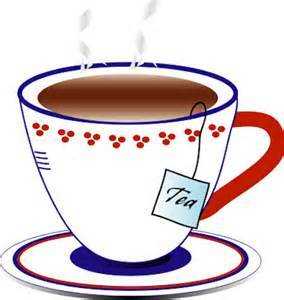# Tea Donations Needed

If you're able to donate individually wrapped tea bags to the teachers lounge, it would be greatly appreciated. Especially popular are herbal, fruit teas, and decaffeinated varieties. They can be dropped off in the main office and should be marked, "Teachers Lounge".

Thank you so much!

 table div table+table+table+table+table+table+table+table+table+table+table+table+table+table+table+table+table+table+table+table+table+table+table+table+table+table+table+table+table+table+table div table{width:100%;padding:0}table div table+table+table+table+table+table+table+table+table+table+table+table+table+table+table+table+table+table+table+table+table+table+table+table+table+table+table+table+table+table+table div table img{width:96.23%;padding:0;float:none}table div table+table+table+table+table+table+table+table+table+table+table+table+table+table+table+table+table+table+table+table+table+table+table+table+table+table+table+table+table+table+table div table td{width:100%;padding:0 1.88% 18px}/* styles */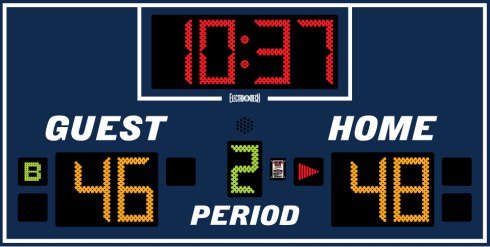# Student or Parent Volunteers Needed for NCP Basketball

Student or parent volunteers are needed to help staff the scorer’s table (operating the new scoreboard and keeping the scorebook) during the regular season and at tournament games for both men's and women's basketball. A small courtesy payment (to come from concessions proceeds) will be offered to interested students. Two volunteers needed per game.

 table div table+table+table+table+table+table+table+table+table+table+table+table+table+table+table+table+table+table+table+table+table+table+table+table+table+table+table+table+table+table+table+table+table div table{width:100%;padding:0}table div table+table+table+table+table+table+table+table+table+table+table+table+table+table+table+table+table+table+table+table+table+table+table+table+table+table+table+table+table+table+table+table+table div table img{width:96.23%;padding:0;float:none}table div table+table+table+table+table+table+table+table+table+table+table+table+table+table+table+table+table+table+table+table+table+table+table+table+table+table+table+table+table+table+table+table+table div table td{width:100%;padding:0 1.88% 18px}/* styles */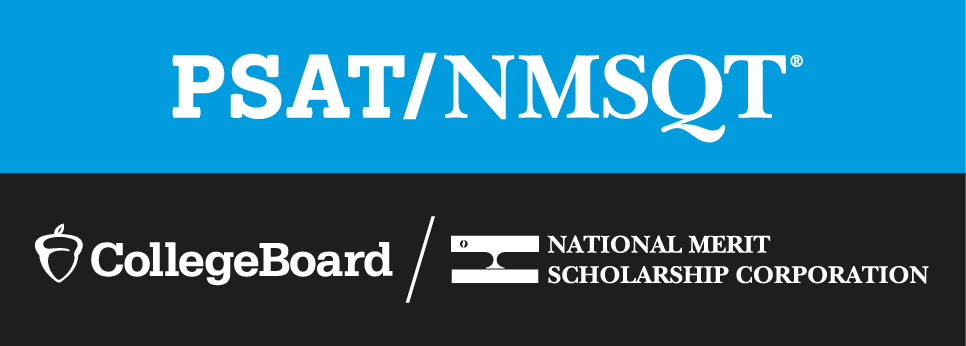# PSAT/NMSQT Update

## 11th Grade Students Will be Able to Submit Their April 2020 SAT Test Scores for Consideration for the National Merit Scholarship

Due to the CTU strike, the PSAT/SAT for sophomores and juniors scheduled for Wednesday, October 30, was cancelled. CPS has confirmation from the College Board and the National Merit Scholarship Corporation that juniors will be able to submit their April 2020 SAT test scores for consideration for the National Merit Scholarship.

 table div table+table+table+table+table+table+table+table+table+table+table+table+table+table+table+table+table+table+table+table+table+table+table+table+table+table+table+table+table+table+table+table+table+table+table+table div table{width:100%;padding:0}table div table+table+table+table+table+table+table+table+table+table+table+table+table+table+table+table+table+table+table+table+table+table+table+table+table+table+table+table+table+table+table+table+table+table+table+table div table img{width:96.23%;padding:0;float:none}table div table+table+table+table+table+table+table+table+table+table+table+table+table+table+table+table+table+table+table+table+table+table+table+table+table+table+table+table+table+table+table+table+table+table+table+table div table td{width:100%;padding:0 1.88% 18px}/* styles */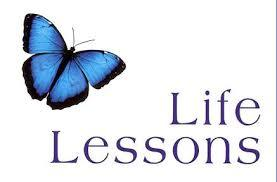# Guest Speakers Needed for Life Lessons Colloquium

Mr. Madia is looking for parents who would like to be a guest speaker for his life lessons colloquium.

He's looking for people in the field of finance, cooking, fashion, travel, hiring manager, auto, housing, life skills, etc. Please contact Mr. Madia directly to help.

 table div table+table+table+table+table+table+table+table+table+table+table+table+table+table+table+table+table+table+table+table+table+table+table+table+table+table+table+table+table+table+table+table+table+table+table+table+table+table div table{width:100%;padding:0}table div table+table+table+table+table+table+table+table+table+table+table+table+table+table+table+table+table+table+table+table+table+table+table+table+table+table+table+table+table+table+table+table+table+table+table+table+table+table div table img{width:96.23%;padding:0;float:none}table div table+table+table+table+table+table+table+table+table+table+table+table+table+table+table+table+table+table+table+table+table+table+table+table+table+table+table+table+table+table+table+table+table+table+table+table+table+table div table td{width:100%;padding:0 1.88% 18px}/* styles */# Donations Needed for Student Resource Closet

Friends of Northside has a resource closet available to NCP students. Currently, we are in need of:

▪ Individual snacks such as granola bars, nuts, and dried fruit
▪ Body lotion
▪ Deodorant for women
▪ Reusable water bottles
▪ Athletic apparel for men and women (shirts, shorts, pants)
▪ Hair conditioner
▪ Men’s and women’s underwear and socks
▪ Mittens and gloves
▪ Sanitizing wipes (Clorox type)
▪ Bath towels
▪ Hair ties
▪ Earmuffs
▪ Baby wipes
 ▪ Individual snacks such as granola bars, nuts, and dried fruit
 ▪ Body lotion
 ▪ Deodorant for women
 ▪ Reusable water bottles
 ▪ Athletic apparel for men and women (shirts, shorts, pants)
 ▪ Hair conditioner
 ▪ Men’s and women’s underwear and socks
 ▪ Mittens and gloves
 ▪ Sanitizing wipes (Clorox type)
 ▪ Bath towels
 ▪ Hair ties
 ▪ Earmuffs
 ▪ Baby wipes

All items must be new and unopened. No used clothing will be accepted. Please bring all items to the Counseling Office and set outside of Ms. Matthews' office.

Contact Nancy Liljedahl with any questions.

 table div table+table+table+table+table+table+table+table+table+table+table+table+table+table+table+table+table+table+table+table+table+table+table+table+table+table+table+table+table+table+table+table+table+table+table+table+table+table+table+table+table div table{width:100%;padding:0}table div table+table+table+table+table+table+table+table+table+table+table+table+table+table+table+table+table+table+table+table+table+table+table+table+table+table+table+table+table+table+table+table+table+table+table+table+table+table+table+table+table div table img{width:96.23%;padding:0;float:none}table div table+table+table+table+table+table+table+table+table+table+table+table+table+table+table+table+table+table+table+table+table+table+table+table+table+table+table+table+table+table+table+table+table+table+table+table+table+table+table+table+table div table td{width:100%;padding:0 1.88% 18px}/* styles */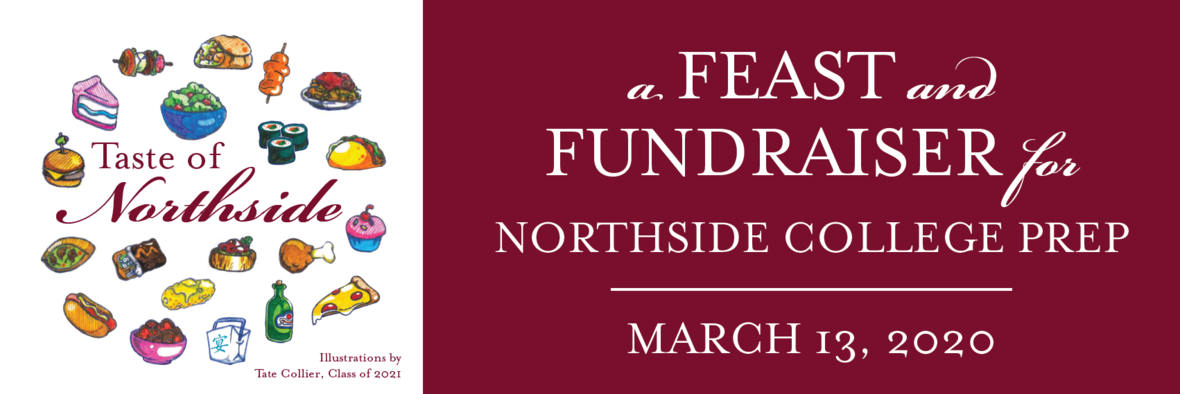# SAVE THE DATE

Friday, March 13, 2020
7:00 - 10:30 pm
DANK Haus, 4740 N Western Ave

Join Northside parents and faculty for a fun evening to raise
essential funding for our students and celebrate cultural diversity with a buffet of local favorites and craft spirits.

## WANT TO HELP?

▪ We need volunteers for videography, decorations, solicitations, and more.
▪ Want more information on how to get involved? Contact Event Chair, Debra Swan.
 ▪ We need volunteers for videography, decorations, solicitations, and more.
 ▪ Want more information on how to get involved? Contact Event Chair, Debra Swan.

## WILLING TO DONATE?

 table div table+table+table+table+table+table+table+table+table+table+table+table+table+table+table+table+table+table+table+table+table+table+table+table+table+table+table+table+table+table+table+table+table+table+table+table+table+table+table+table+table+table+table+table div table{width:100%;padding:0}table div table+table+table+table+table+table+table+table+table+table+table+table+table+table+table+table+table+table+table+table+table+table+table+table+table+table+table+table+table+table+table+table+table+table+table+table+table+table+table+table+table+table+table+table div table img{width:96.23%;padding:0;float:none}table div table+table+table+table+table+table+table+table+table+table+table+table+table+table+table+table+table+table+table+table+table+table+table+table+table+table+table+table+table+table+table+table+table+table+table+table+table+table+table+table+table+table+table+table div table td{width:100%;padding:0 1.88% 18px}/* styles */# Band Trip to Orlando for Festival Disney

Thursday, March 12-Sunday, March 15, 2020

All non-beginning level members of Band, Jazz Band, Orchestra, and Choir are invited to travel to Orlando to participate in Festival Disney, a music performance competition and festival for high school music ensembles from across the country, from Thursday, March 12 through Sunday, March 15, 2020.

1. Read through the trip details.
2. If you are committed to participating, complete and submit the commitment form. COMMITMENT FORM
3. Officially register for the trip and begin your payments by way of the online registration and payment portal.
 1 Read through the trip details.
 2 If you are committed to participating, complete and submit the commitment form. COMMITMENT FORM
 3 Officially register for the trip and begin your payments by way of the online registration and payment portal.

Contact Mr. Park if you have any questions.

 table div table+table+table+table+table+table+table+table+table+table+table+table+table+table+table+table+table+table+table+table+table+table+table+table+table+table+table+table+table+table+table+table+table+table+table+table+table+table+table+table+table+table+table+table+table+table+table div table{width:100%;padding:0}table div table+table+table+table+table+table+table+table+table+table+table+table+table+table+table+table+table+table+table+table+table+table+table+table+table+table+table+table+table+table+table+table+table+table+table+table+table+table+table+table+table+table+table+table+table+table+table div table img{width:96.23%;padding:0;float:none}table div table+table+table+table+table+table+table+table+table+table+table+table+table+table+table+table+table+table+table+table+table+table+table+table+table+table+table+table+table+table+table+table+table+table+table+table+table+table+table+table+table+table+table+table+table+table+table div table td{width:100%;padding:0 1.88% 18px}/* styles */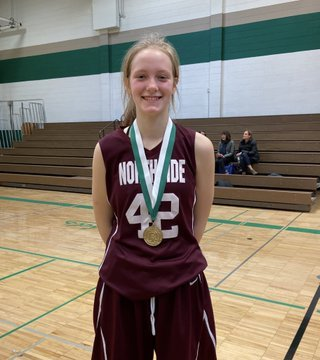# NCP Freshmen Named to Ridgewood All-Tournament Team

Congratulations to Northside freshman Cate Shingler on being named to the Ridgewood Girl’s Basketball Tournament’s all-tournament team. She is the second NCP freshman to be named to the team following Alina Yamin back in 2015.

# Do you have news or team results to share with the Northside parent community?

 table div table+table+table+table+table+table+table+table+table+table+table+table+table+table+table+table+table+table+table+table+table+table+table+table+table+table+table+table+table+table+table+table+table+table+table+table+table+table+table+table+table+table+table+table+table+table+table+table+table+table+table div table{width:100%;padding:0}table div table+table+table+table+table+table+table+table+table+table+table+table+table+table+table+table+table+table+table+table+table+table+table+table+table+table+table+table+table+table+table+table+table+table+table+table+table+table+table+table+table+table+table+table+table+table+table+table+table+table+table div table img{width:96.23%;padding:0;float:none}table div table+table+table+table+table+table+table+table+table+table+table+table+table+table+table+table+table+table+table+table+table+table+table+table+table+table+table+table+table+table+table+table+table+table+table+table+table+table+table+table+table+table+table+table+table+table+table+table+table+table+table div table td{width:100%;padding:0 1.88% 18px}/* styles */# November/Early December College Visits at Northside

Monday, December 2
Lincoln Technical Institute-Chicago, 10:38 am

Tuesday, December 3
National Louis University-Chicago Campus, 11:36 am

Students can register for the upcoming visits via Naviance or the Enriching Student Portal. Parents can access the college visits via their Parent Naviance account.

 table div table+table+table+table+table+table+table+table+table+table+table+table+table+table+table+table+table+table+table+table+table+table+table+table+table+table+table+table+table+table+table+table+table+table+table+table+table+table+table+table+table+table+table+table+table+table+table+table+table+table+table+table+table+table div table{width:100%;padding:0}table div table+table+table+table+table+table+table+table+table+table+table+table+table+table+table+table+table+table+table+table+table+table+table+table+table+table+table+table+table+table+table+table+table+table+table+table+table+table+table+table+table+table+table+table+table+table+table+table+table+table+table+table+table+table div table img{width:96.23%;padding:0;float:none}table div table+table+table+table+table+table+table+table+table+table+table+table+table+table+table+table+table+table+table+table+table+table+table+table+table+table+table+table+table+table+table+table+table+table+table+table+table+table+table+table+table+table+table+table+table+table+table+table+table+table+table+table+table+table div table td{width:100%;padding:0 1.88% 18px}/* styles */# Collegiate Scholars Information Session (9th Grade Only)

Wednesday, December 4
2:15 - 3:00 pm
NCP Cafeteria

A representative from The University of Chicago Collegiate Scholars Program (CSP) will conduct an information session for 9th-grade students. If you are interested in participating in the information session, register via the Enriching Student Portal for the Collegiate Scholars Information during Flex Block 4 by Tuesday, December 2.

Chicago Collegiate Scholars is a three-year enrichment program for academically successful and highly motivated Chicago Public High School students. CSP prepares students for admission and success at the nation's most selective colleges and universities. The program is currently accepting applications for the Class of 2023.

 table div table+table+table+table+table+table+table+table+table+table+table+table+table+table+table+table+table+table+table+table+table+table+table+table+table+table+table+table+table+table+table+table+table+table+table+table+table+table+table+table+table+table+table+table+table+table+table+table+table+table+table+table+table+table+table+table div table{width:100%;padding:0}table div table+table+table+table+table+table+table+table+table+table+table+table+table+table+table+table+table+table+table+table+table+table+table+table+table+table+table+table+table+table+table+table+table+table+table+table+table+table+table+table+table+table+table+table+table+table+table+table+table+table+table+table+table+table+table+table div table img{width:96.23%;padding:0;float:none}table div table+table+table+table+table+table+table+table+table+table+table+table+table+table+table+table+table+table+table+table+table+table+table+table+table+table+table+table+table+table+table+table+table+table+table+table+table+table+table+table+table+table+table+table+table+table+table+table+table+table+table+table+table+table+table+table div table td{width:100%;padding:0 1.88% 18px}/* styles */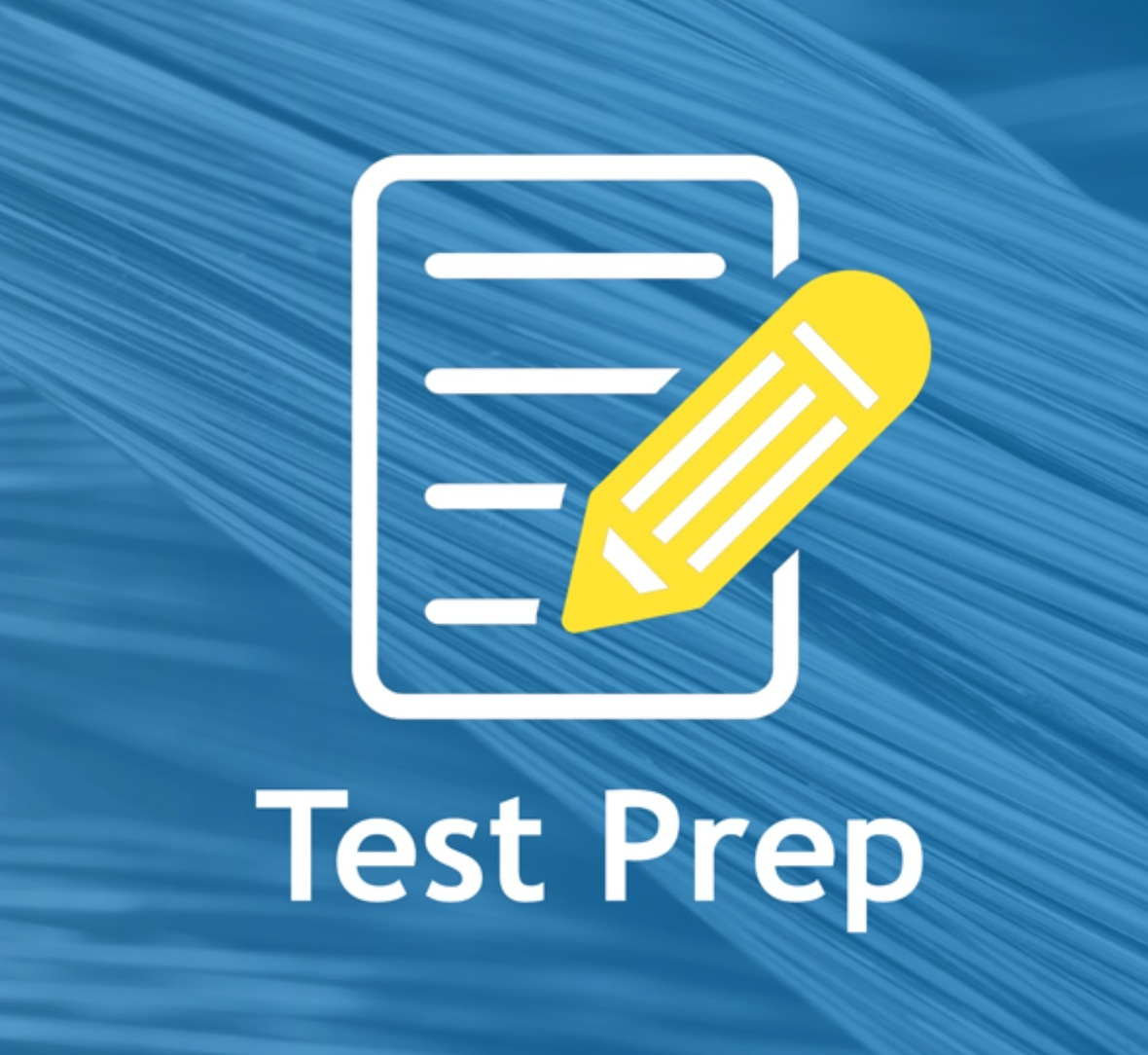# Academic Approach SAT Test Prep Course

Academic Approach is offering a standardized test preparation course for students taking the April 14, 2020, SAT exam. This course will review key skills in reading, writing, math, and science and their application on the SAT.

Cost: \$356.40 per student.
Class Time: Wednesdays 2:15 pm – 5:15 pm
Deadline to register: January 6, 2020

 table div table+table+table+table+table+table+table+table+table+table+table+table+table+table+table+table+table+table+table+table+table+table+table+table+table+table+table+table+table+table+table+table+table+table+table+table+table+table+table+table+table+table+table+table+table+table+table+table+table+table+table+table+table+table+table+table+table+table div table{width:100%;padding:0}table div table+table+table+table+table+table+table+table+table+table+table+table+table+table+table+table+table+table+table+table+table+table+table+table+table+table+table+table+table+table+table+table+table+table+table+table+table+table+table+table+table+table+table+table+table+table+table+table+table+table+table+table+table+table+table+table+table+table div table img{width:96.23%;padding:0;float:none}table div table+table+table+table+table+table+table+table+table+table+table+table+table+table+table+table+table+table+table+table+table+table+table+table+table+table+table+table+table+table+table+table+table+table+table+table+table+table+table+table+table+table+table+table+table+table+table+table+table+table+table+table+table+table+table+table+table+table div table td{width:100%;padding:0 1.88% 18px}/* styles */# CPS Scholarship Notifications and College Events Around the City

For details about the upcoming events and to access the most recent updates, view the College Calendar on the Northside Prep Counseling website HERE.

Also, for additional scholarship opportunities visit the Northside College Prep Counseling page HERE

 table div table+table+table+table+table+table+table+table+table+table+table+table+table+table+table+table+table+table+table+table+table+table+table+table+table+table+table+table+table+table+table+table+table+table+table+table+table+table+table+table+table+table+table+table+table+table+table+table+table+table+table+table+table+table+table+table+table+table+table+table div table{width:100%;padding:0}table div table+table+table+table+table+table+table+table+table+table+table+table+table+table+table+table+table+table+table+table+table+table+table+table+table+table+table+table+table+table+table+table+table+table+table+table+table+table+table+table+table+table+table+table+table+table+table+table+table+table+table+table+table+table+table+table+table+table+table+table div table img{width:96.23%;padding:0;float:none}table div table+table+table+table+table+table+table+table+table+table+table+table+table+table+table+table+table+table+table+table+table+table+table+table+table+table+table+table+table+table+table+table+table+table+table+table+table+table+table+table+table+table+table+table+table+table+table+table+table+table+table+table+table+table+table+table+table+table+table+table div table td{width:100%;padding:0 1.88% 18px}/* styles */## Important Dates for the Class of 2020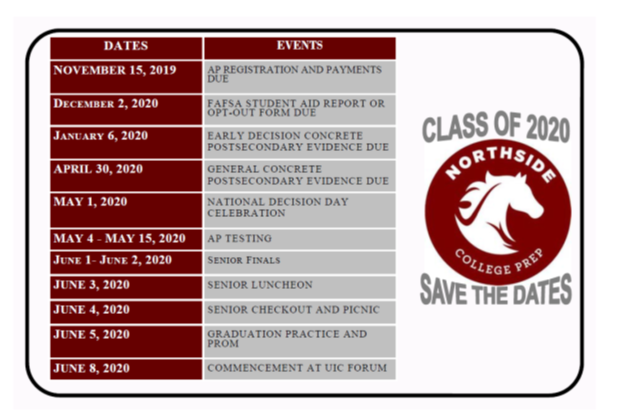## Important Dates for the Class of 2021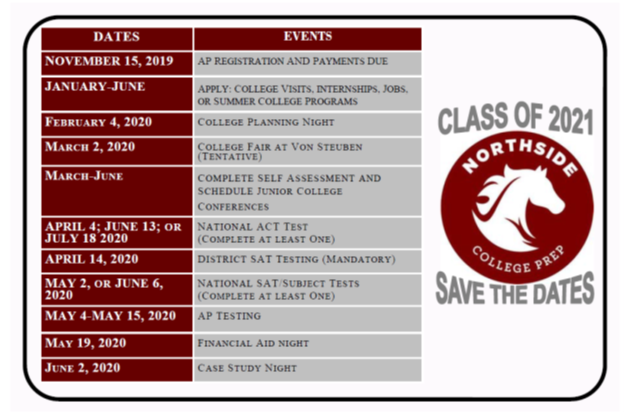table div table+table+table+table+table+table+table+table+table+table+table+table+table+table+table+table+table+table+table+table+table+table+table+table+table+table+table+table+table+table+table+table+table+table+table+table+table+table+table+table+table+table+table+table+table+table+table+table+table+table+table+table+table+table+table+table+table+table+table+table+table+table+table+table+table div table{width:100%;padding:0}table div table+table+table+table+table+table+table+table+table+table+table+table+table+table+table+table+table+table+table+table+table+table+table+table+table+table+table+table+table+table+table+table+table+table+table+table+table+table+table+table+table+table+table+table+table+table+table+table+table+table+table+table+table+table+table+table+table+table+table+table+table+table+table+table+table div table img{width:96.23%;padding:0;float:none}table div table+table+table+table+table+table+table+table+table+table+table+table+table+table+table+table+table+table+table+table+table+table+table+table+table+table+table+table+table+table+table+table+table+table+table+table+table+table+table+table+table+table+table+table+table+table+table+table+table+table+table+table+table+table+table+table+table+table+table+table+table+table+table+table+table div table td{width:100%;padding:0 1.88% 18px}/* styles */# New to Parent Portal?

1. Obtain your student's PIN, which should be on your acceptance letter or school welcome letter.
2. Create an account HERE.
 1 Obtain your student's PIN, which should be on your acceptance letter or school welcome letter.
 2 Create an account HERE.

PINs can only be provided to the parent/guardian in person or by mail to the address on file. They cannot be provided by phone or email, or to a student. To request your PIN, please contact Sonia Fernandez in the Counseling Office.

 table div table+table+table+table+table+table+table+table+table+table+table+table+table+table+table+table+table+table+table+table+table+table+table+table+table+table+table+table+table+table+table+table+table+table+table+table+table+table+table+table+table+table+table+table+table+table+table+table+table+table+table+table+table+table+table+table+table+table+table+table+table+table+table+table+table+table+table div table{width:100%;padding:0}table div table+table+table+table+table+table+table+table+table+table+table+table+table+table+table+table+table+table+table+table+table+table+table+table+table+table+table+table+table+table+table+table+table+table+table+table+table+table+table+table+table+table+table+table+table+table+table+table+table+table+table+table+table+table+table+table+table+table+table+table+table+table+table+table+table+table+table div table img{width:96.23%;padding:0;float:none}table div table+table+table+table+table+table+table+table+table+table+table+table+table+table+table+table+table+table+table+table+table+table+table+table+table+table+table+table+table+table+table+table+table+table+table+table+table+table+table+table+table+table+table+table+table+table+table+table+table+table+table+table+table+table+table+table+table+table+table+table+table+table+table+table+table+table+table div table td{width:100%;padding:0 1.88% 18px}/* styles */# CPS Volunteer Requirements

According to CPS policy, anybody who is going to be in contact with students must be approved as a CPS volunteer. There are two levels of volunteers, Level 1 and Level 2.

## Level I Volunteers

(Please allow 8-12 weeks for the entire process)
A fingerprint criminal background check is required for all Level I Volunteers.

A Level I volunteer is:

1. A parent providing volunteer service in their child’s school or classroom for 10 or more hours/week;
2. An individual providing volunteer service for 5 or more hours/week at a school where s/he does not have a child enrolled;
3. Any individual chaperoning an overnight school-sponsored trip, regardless of the hours/week that the volunteer serves (See also the Student Travel Policy).
 1 A parent providing volunteer service in their child’s school or classroom for 10 or more hours/week;
 2 An individual providing volunteer service for 5 or more hours/week at a school where s/he does not have a child enrolled;
 3 Any individual chaperoning an overnight school-sponsored trip, regardless of the hours/week that the volunteer serves (See also the Student Travel Policy).

## Level II Volunteers

(This process can be completed in a day & is required even of volunteers for Friends of Northside events)
A fingerprint criminal background check is not required for Level II Volunteers.

A Level II Volunteer is:

1. A parent providing supervised volunteer service in their child’s school or classroom for less than 10 hours/week;
2. Any individual providing volunteer service for less than 5 hours/week at a school where s/he does not have a child enrolled;
3. An individual providing incidental volunteer service with no ongoing individualized interaction with a student(s) including those who speak at a class/assembly, judge academic competitions, give musical performance, participate in the “Principal for a Day” program, job shadowing event or other one-time event provided where there is direct supervision of the activity/event by regular school employees;
4. A parent accompanying his/her child’s class on a one-day field trip or another type of extracurricular activity that does not involve an overnight stay;
5. An individual providing volunteer service on projects/activities involving no or nominal contact with children (home-based volunteer activities, volunteers serving at Central or Network office).
 1 A parent providing supervised volunteer service in their child’s school or classroom for less than 10 hours/week;
 2 Any individual providing volunteer service for less than 5 hours/week at a school where s/he does not have a child enrolled;
 3 An individual providing incidental volunteer service with no ongoing individualized interaction with a student(s) including those who speak at a class/assembly, judge academic competitions, give musical performance, participate in the “Principal for a Day” program, job shadowing event or other one-time event provided where there is direct supervision of the activity/event by regular school employees;
 4 A parent accompanying his/her child’s class on a one-day field trip or another type of extracurricular activity that does not involve an overnight stay;
 5 An individual providing volunteer service on projects/activities involving no or nominal contact with children (home-based volunteer activities, volunteers serving at Central or Network office).

To become a volunteer, please visit CPS' Volunteer website and follow the instructions. There is also a link on Northside's website on the Parents page Northside Volunteers.

Assistant Principal, Kaitlin O'Hare is Northside's Volunteer Coordinator. After applying to become a volunteer, you must visit the school and show Ms. O'Hare your ID, so she can approve you to move forward in the volunteer process. For Level II volunteers, that is the only step you must take. For Level 1, you will receive further instructions from CPS.

 table div table+table+table+table+table+table+table+table+table+table+table+table+table+table+table+table+table+table+table+table+table+table+table+table+table+table+table+table+table+table+table+table+table+table+table+table+table+table+table+table+table+table+table+table+table+table+table+table+table+table+table+table+table+table+table+table+table+table+table+table+table+table+table+table+table+table+table+table+table div table{width:100%;padding:0}table div table+table+table+table+table+table+table+table+table+table+table+table+table+table+table+table+table+table+table+table+table+table+table+table+table+table+table+table+table+table+table+table+table+table+table+table+table+table+table+table+table+table+table+table+table+table+table+table+table+table+table+table+table+table+table+table+table+table+table+table+table+table+table+table+table+table+table+table+table div table img{width:96.23%;padding:0;float:none}table div table+table+table+table+table+table+table+table+table+table+table+table+table+table+table+table+table+table+table+table+table+table+table+table+table+table+table+table+table+table+table+table+table+table+table+table+table+table+table+table+table+table+table+table+table+table+table+table+table+table+table+table+table+table+table+table+table+table+table+table+table+table+table+table+table+table+table+table+table div table td{width:100%;padding:0 1.88% 18px}/* styles */# FON Connect

Friends of Northside uses a customer/constituent relationship management (CRM) system known as FON Connect that allows our parent volunteer organization to work from one database for events. It is not a CPS database and is completely different and separate from the CPS Parent Portal and Northside's EPay website.

FON Connect also serves as the NCP Family Directory and allows users to review their donation history, update their contact information, register for upcoming events, and more. Each parent has a separate account. Transactions, including donations, are recorded in the account of the parent who initiated the transaction or donation.

If you’re new to FON Connect, you'll need to set up a login and password. To do so:

 table div table+table+table+table+table+table+table+table+table+table+table+table+table+table+table+table+table+table+table+table+table+table+table+table+table+table+table+table+table+table+table+table+table+table+table+table+table+table+table+table+table+table+table+table+table+table+table+table+table+table+table+table+table+table+table+table+table+table+table+table+table+table+table+table+table+table+table+table+table+table+table div table{width:100%;padding:0}table div table+table+table+table+table+table+table+table+table+table+table+table+table+table+table+table+table+table+table+table+table+table+table+table+table+table+table+table+table+table+table+table+table+table+table+table+table+table+table+table+table+table+table+table+table+table+table+table+table+table+table+table+table+table+table+table+table+table+table+table+table+table+table+table+table+table+table+table+table+table+table div table img{width:96.23%;padding:0;float:none}table div table+table+table+table+table+table+table+table+table+table+table+table+table+table+table+table+table+table+table+table+table+table+table+table+table+table+table+table+table+table+table+table+table+table+table+table+table+table+table+table+table+table+table+table+table+table+table+table+table+table+table+table+table+table+table+table+table+table+table+table+table+table+table+table+table+table+table+table+table+table+table div table td{width:100%;padding:0 1.88% 18px}/* styles */# Parking Lot Safety

Our NCP school parking lot is a bustling place before and after school. Families dropping off and picking up students are asked to be mindful of both safety and courtesy. Please assist us by ensuring that traffic lanes stay clear during drop-off and pick-up times and by following the parking lot procedures.

# Parking Lot Procedures

ALL vehicles MUST exit onto Kedzie. You may enter the parking lot from Kedzie or Bryn Mawr, but regardless of where you enter, all cars must exit onto Kedzie. For the safety of our students and the rest of the Northside community, please do not exit onto Bryn Mawr.

The first few days will be busy at drop-off and pick-up. Please, for the safety of all involved, plan to give yourself enough time for drop-off and pick-up and please be patient while in the parking lot. Pulling over to the curb on the right as your student enters or exits your vehicle keeps students safe and traffic flow efficient. Our safety team will be actively working to ensure that students arrive and depart safely each day and that vehicles in school lots remain damage-free.

 table div table+table+table+table+table+table+table+table+table+table+table+table+table+table+table+table+table+table+table+table+table+table+table+table+table+table+table+table+table+table+table+table+table+table+table+table+table+table+table+table+table+table+table+table+table+table+table+table+table+table+table+table+table+table+table+table+table+table+table+table+table+table+table+table+table+table+table+table+table+table+table+table+table div table{width:100%;padding:0}table div table+table+table+table+table+table+table+table+table+table+table+table+table+table+table+table+table+table+table+table+table+table+table+table+table+table+table+table+table+table+table+table+table+table+table+table+table+table+table+table+table+table+table+table+table+table+table+table+table+table+table+table+table+table+table+table+table+table+table+table+table+table+table+table+table+table+table+table+table+table+table+table+table div table img{width:96.23%;padding:0;float:none}table div table+table+table+table+table+table+table+table+table+table+table+table+table+table+table+table+table+table+table+table+table+table+table+table+table+table+table+table+table+table+table+table+table+table+table+table+table+table+table+table+table+table+table+table+table+table+table+table+table+table+table+table+table+table+table+table+table+table+table+table+table+table+table+table+table+table+table+table+table+table+table+table+table div table td{width:100%;padding:0 1.88% 18px}/* styles */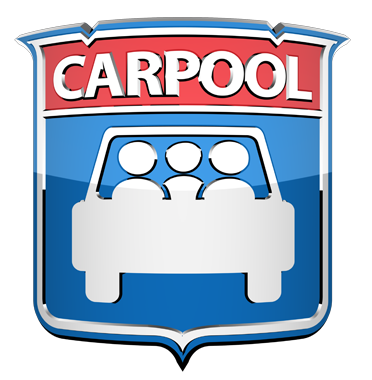# NCP Carpool Connection!

If you're interested in carpooling to school, add your address to the 2019-2020 NCP Carpool map and reach out to contact a potential carpool family on the map. Find the map HERE or on the Welcome page of FON Connect.

 table div table+table+table+table+table+table+table+table+table+table+table+table+table+table+table+table+table+table+table+table+table+table+table+table+table+table+table+table+table+table+table+table+table+table+table+table+table+table+table+table+table+table+table+table+table+table+table+table+table+table+table+table+table+table+table+table+table+table+table+table+table+table+table+table+table+table+table+table+table+table+table+table+table+table+table div table{width:100%;padding:0}table div table+table+table+table+table+table+table+table+table+table+table+table+table+table+table+table+table+table+table+table+table+table+table+table+table+table+table+table+table+table+table+table+table+table+table+table+table+table+table+table+table+table+table+table+table+table+table+table+table+table+table+table+table+table+table+table+table+table+table+table+table+table+table+table+table+table+table+table+table+table+table+table+table+table+table div table img{width:96.23%;padding:0;float:none}table div table+table+table+table+table+table+table+table+table+table+table+table+table+table+table+table+table+table+table+table+table+table+table+table+table+table+table+table+table+table+table+table+table+table+table+table+table+table+table+table+table+table+table+table+table+table+table+table+table+table+table+table+table+table+table+table+table+table+table+table+table+table+table+table+table+table+table+table+table+table+table+table+table+table+table div table td{width:100%;padding:0 1.88% 18px}/* styles */# Attention, Parents of CTA Commuters

Did you know that the #82 and #92 buses pick up students in front of Northside at the end of the school day? The Kimball Brown line station is also close to school at Kimball and Lawrence.

These are just a few of the ways students can use public transportation to commute to school. More information on routes for your student can be found at the CTA website HERE.

 table div table+table+table+table+table+table+table+table+table+table+table+table+table+table+table+table+table+table+table+table+table+table+table+table+table+table+table+table+table+table+table+table+table+table+table+table+table+table+table+table+table+table+table+table+table+table+table+table+table+table+table+table+table+table+table+table+table+table+table+table+table+table+table+table+table+table+table+table+table+table+table+table+table+table+table+table+table div table{width:100%;padding:0}table div table+table+table+table+table+table+table+table+table+table+table+table+table+table+table+table+table+table+table+table+table+table+table+table+table+table+table+table+table+table+table+table+table+table+table+table+table+table+table+table+table+table+table+table+table+table+table+table+table+table+table+table+table+table+table+table+table+table+table+table+table+table+table+table+table+table+table+table+table+table+table+table+table+table+table+table+table div table img{width:96.23%;padding:0;float:none}table div table+table+table+table+table+table+table+table+table+table+table+table+table+table+table+table+table+table+table+table+table+table+table+table+table+table+table+table+table+table+table+table+table+table+table+table+table+table+table+table+table+table+table+table+table+table+table+table+table+table+table+table+table+table+table+table+table+table+table+table+table+table+table+table+table+table+table+table+table+table+table+table+table+table+table+table+table div table td{width:100%;padding:0 1.88% 18px}/* styles */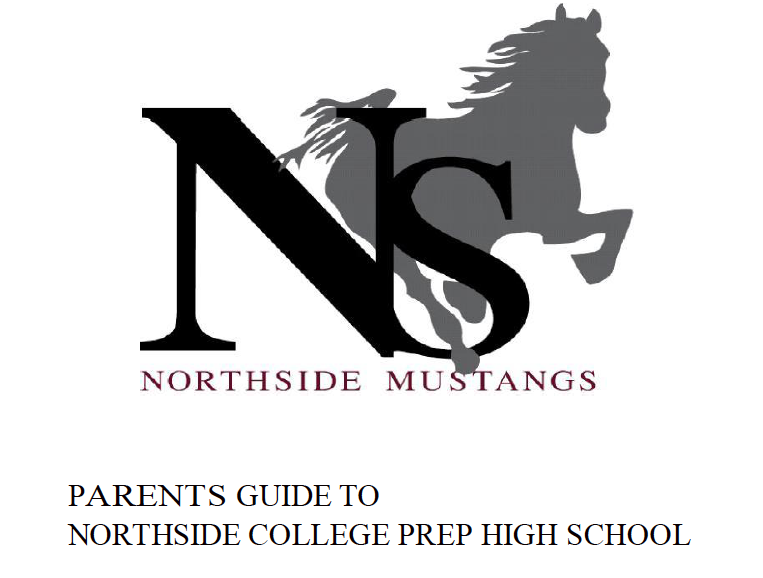# Navigating Northside

Navigating Northside, our parent handbook, is available on the school website under the "Parents" tab. Click HERE to access it directly.

 table div table+table+table+table+table+table+table+table+table+table+table+table+table+table+table+table+table+table+table+table+table+table+table+table+table+table+table+table+table+table+table+table+table+table+table+table+table+table+table+table+table+table+table+table+table+table+table+table+table+table+table+table+table+table+table+table+table+table+table+table+table+table+table+table+table+table+table+table+table+table+table+table+table+table+table+table+table+table+table div table{width:100%;padding:0}table div table+table+table+table+table+table+table+table+table+table+table+table+table+table+table+table+table+table+table+table+table+table+table+table+table+table+table+table+table+table+table+table+table+table+table+table+table+table+table+table+table+table+table+table+table+table+table+table+table+table+table+table+table+table+table+table+table+table+table+table+table+table+table+table+table+table+table+table+table+table+table+table+table+table+table+table+table+table+table div table img{width:96.23%;padding:0;float:none}table div table+table+table+table+table+table+table+table+table+table+table+table+table+table+table+table+table+table+table+table+table+table+table+table+table+table+table+table+table+table+table+table+table+table+table+table+table+table+table+table+table+table+table+table+table+table+table+table+table+table+table+table+table+table+table+table+table+table+table+table+table+table+table+table+table+table+table+table+table+table+table+table+table+table+table+table+table+table+table div table td{width:100%;padding:0 1.88% 18px}/* styles */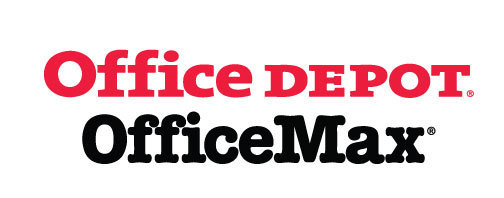# Give Back to Northside

Purchase school and office supplies at Office Depot and OfficeMax and Northside can receive 5% back in credits or FREE supplies. Simply provide our school ID 70031171 at checkout, online, or in stores. There’s no cost to you!

 table div table+table+table+table+table+table+table+table+table+table+table+table+table+table+table+table+table+table+table+table+table+table+table+table+table+table+table+table+table+table+table+table+table+table+table+table+table+table+table+table+table+table+table+table+table+table+table+table+table+table+table+table+table+table+table+table+table+table+table+table+table+table+table+table+table+table+table+table+table+table+table+table+table+table+table+table+table+table+table+table+table div table{width:100%;padding:0}table div table+table+table+table+table+table+table+table+table+table+table+table+table+table+table+table+table+table+table+table+table+table+table+table+table+table+table+table+table+table+table+table+table+table+table+table+table+table+table+table+table+table+table+table+table+table+table+table+table+table+table+table+table+table+table+table+table+table+table+table+table+table+table+table+table+table+table+table+table+table+table+table+table+table+table+table+table+table+table+table+table div table img{width:96.23%;padding:0;float:none}table div table+table+table+table+table+table+table+table+table+table+table+table+table+table+table+table+table+table+table+table+table+table+table+table+table+table+table+table+table+table+table+table+table+table+table+table+table+table+table+table+table+table+table+table+table+table+table+table+table+table+table+table+table+table+table+table+table+table+table+table+table+table+table+table+table+table+table+table+table+table+table+table+table+table+table+table+table+table+table+table+table div table td{width:100%;padding:0 1.88% 18px}/* styles */# Support Northside - Shop AmazonSmile

AmazonSmile is a simple and automatic way to support Northside every time you shop.

Please note that our AmazonSmile name is Friends of "North" "Side" (North Side - two words at AmazonSmile).

 table div table+table+table+table+table+table+table+table+table+table+table+table+table+table+table+table+table+table+table+table+table+table+table+table+table+table+table+table+table+table+table+table+table+table+table+table+table+table+table+table+table+table+table+table+table+table+table+table+table+table+table+table+table+table+table+table+table+table+table+table+table+table+table+table+table+table+table+table+table+table+table+table+table+table+table+table+table+table+table+table+table+table+table div table{width:100%;padding:0}table div table+table+table+table+table+table+table+table+table+table+table+table+table+table+table+table+table+table+table+table+table+table+table+table+table+table+table+table+table+table+table+table+table+table+table+table+table+table+table+table+table+table+table+table+table+table+table+table+table+table+table+table+table+table+table+table+table+table+table+table+table+table+table+table+table+table+table+table+table+table+table+table+table+table+table+table+table+table+table+table+table+table+table div table img{width:96.23%;padding:0;float:none}table div table+table+table+table+table+table+table+table+table+table+table+table+table+table+table+table+table+table+table+table+table+table+table+table+table+table+table+table+table+table+table+table+table+table+table+table+table+table+table+table+table+table+table+table+table+table+table+table+table+table+table+table+table+table+table+table+table+table+table+table+table+table+table+table+table+table+table+table+table+table+table+table+table+table+table+table+table+table+table+table+table+table+table div table td{width:100%;padding:0 1.88% 18px}/* styles */# Follow Us on Social Media

▪ Northside Prep Parents Facebook Page, a closed group for parents, by parents, to share their knowledge of Northside
▪ Friends of Northside Facebook Page, for news everyone can view
 ▪ Northside Prep Parents Facebook Page, a closed group for parents, by parents, to share their knowledge of Northside
 ▪ Friends of Northside Facebook Page, for news everyone can view
 table div table+table+table+table+table+table+table+table+table+table+table+table+table+table+table+table+table+table+table+table+table+table+table+table+table+table+table+table+table+table+table+table+table+table+table+table+table+table+table+table+table+table+table+table+table+table+table+table+table+table+table+table+table+table+table+table+table+table+table+table+table+table+table+table+table+table+table+table+table+table+table+table+table+table+table+table+table+table+table+table+table+table+table+table+table div table{width:100%;padding:0}table div table+table+table+table+table+table+table+table+table+table+table+table+table+table+table+table+table+table+table+table+table+table+table+table+table+table+table+table+table+table+table+table+table+table+table+table+table+table+table+table+table+table+table+table+table+table+table+table+table+table+table+table+table+table+table+table+table+table+table+table+table+table+table+table+table+table+table+table+table+table+table+table+table+table+table+table+table+table+table+table+table+table+table+table+table div table img{width:96.23%;padding:0;float:none}table div table+table+table+table+table+table+table+table+table+table+table+table+table+table+table+table+table+table+table+table+table+table+table+table+table+table+table+table+table+table+table+table+table+table+table+table+table+table+table+table+table+table+table+table+table+table+table+table+table+table+table+table+table+table+table+table+table+table+table+table+table+table+table+table+table+table+table+table+table+table+table+table+table+table+table+table+table+table+table+table+table+table+table+table+table div table td{width:100%;padding:0 1.88% 18px}/* styles */Northside’s website can be found HERE.

Friends of Northside's website can be found HERE.

 table div table+table+table+table+table+table+table+table+table+table+table+table+table+table+table+table+table+table+table+table+table+table+table+table+table+table+table+table+table+table+table+table+table+table+table+table+table+table+table+table+table+table+table+table+table+table+table+table+table+table+table+table+table+table+table+table+table+table+table+table+table+table+table+table+table+table+table+table+table+table+table+table+table+table+table+table+table+table+table+table+table+table+table+table+table+table+table div table{width:100%;padding:0}table div table+table+table+table+table+table+table+table+table+table+table+table+table+table+table+table+table+table+table+table+table+table+table+table+table+table+table+table+table+table+table+table+table+table+table+table+table+table+table+table+table+table+table+table+table+table+table+table+table+table+table+table+table+table+table+table+table+table+table+table+table+table+table+table+table+table+table+table+table+table+table+table+table+table+table+table+table+table+table+table+table+table+table+table+table+table+table div table img{width:96.23%;padding:0;float:none}table div table+table+table+table+table+table+table+table+table+table+table+table+table+table+table+table+table+table+table+table+table+table+table+table+table+table+table+table+table+table+table+table+table+table+table+table+table+table+table+table+table+table+table+table+table+table+table+table+table+table+table+table+table+table+table+table+table+table+table+table+table+table+table+table+table+table+table+table+table+table+table+table+table+table+table+table+table+table+table+table+table+table+table+table+table+table+table div table td{width:100%;padding:0 1.88% 18px}/* styles */